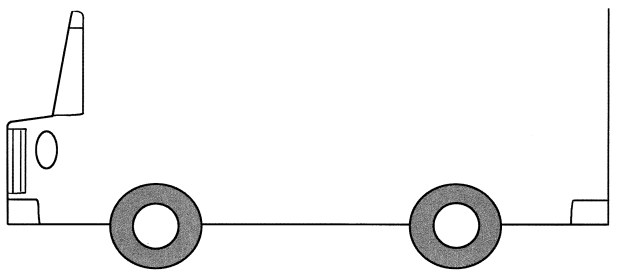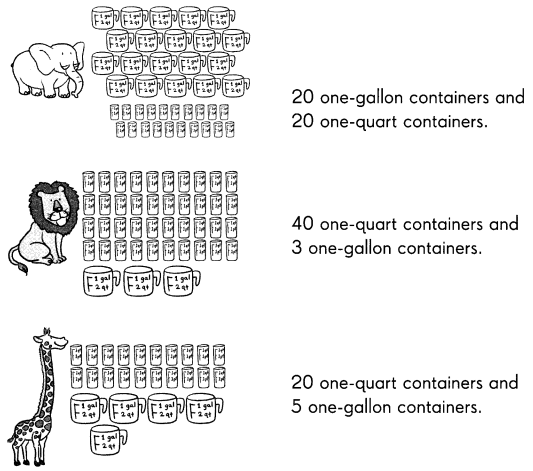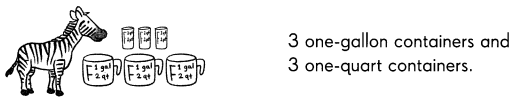# Math in Focus Grade 3 Chapter 15 Answer Key Customary Length, Weight, and Capacity

This handy Math in Focus Grade 3 Workbook Answer Key Chapter 15 Customary Length, Weight, and Capacity provides detailed solutions for the textbook questions.

## Math in Focus Grade 3 Chapter 15 Answer Key Customary Length, Weight, and Capacity

Challenging Practice

Question 1.
Follow the directions to complete the drawing.
Step 1: Join the top of the lines at each end of the bus.
Step 2: Find the length of the bus in inches.
Step 3: Draw 3________ square windows. Each has sides that are $$\frac{1}{2}$$ inch long.
Step 4: The windows are $$\frac{1}{2}$$inch from the top of the bus.
Step 5: Draw a door between the 2 wheels. The height of the door is 1 inch. The width is 1 inch.
Step 6: Find the height from the top of the windows to the bottom of the bus to the nearest inch.
Step 7: Color and decorate your bus.Problem Solving

• The veterinarian at City Zoo keeps track of how much water each animal drinks in a period of time. Decide which animal drinks the greatest amount of water and fill in the blank.The ___________ drinks the greatest amount of water.
The elephant drinks the greatest amount of water.
Explanation:
We know that 1 pint is equal to 2 cups.
1 quarts is equal to 2 pints.
1 gallon is equal to 4 quarts.
• The elephant drinks 20 one – gallon containers and 20 one – quart containers.
20/4 = 5 gallons
20 gallons + 5 gallons = 25 gallons
The elephant drinks 25 gallons of water.
• The lion drinks 40 one – quarts containers and 3 one – gallon containers.
40/4 = 10 gallons
10 gallons + 3 gallons = 13 gallons
The lion drinks 13 gallons of water.
• The Giraffe drinks 20 one – quart containers and 5 one – gallon containers.
20/4 = 5 gallons
5 gallons + 5 gallons = 10 gallons
The giraffe drinks 10 gallons of water.
• The zebra drinks 3 one – gallon containers and 3 one – quart containers.
3 gallons and 3 quarts is slightly greater than 3 gallons.
The zebra drinks 3 gallons of water.